# dempk

dempk performs a merging of components found by tkmeans

## Syntax

• out=dempk(Y, k, g)example
• out=dempk(Y, k, g,Name,Value)example

## Description

The function dempk performs either a hierarchical merging of the k components found by tkmeans (using the pairwise overlap values between them and giving g clusters), or if g is a decimal number between 0 and 1 it performs the merging phase according to the threshold g (the same algorithm as overlapmap).

 out =dempk(Y, k, g) Example using dempk on data obtained by simdataset, specifying both hierarchical clustering and a threshold value, in order to obtain additional plots.

 out =dempk(Y, k, g, Name, Value) Example using dempk with hierarchical merging on data obtained by simdataset, specifying additional arguments in the call to tkmeans.

## Examples

expand all

###Example using dempk on data obtained by simdataset, specifying both hierarchical clustering and a threshold value, in order to obtain additional plots.

close all
% Specify k cluster in v dimensions with n obs
k = 10;
v = 2;
n = 5000;
% Generate homogeneous and spherical clusters
rng(100, 'twister');
out = MixSim(k, v, 'sph', true, 'hom', true, 'int', [0 10], 'Display', 'off', 'BarOmega', 0.05, 'Display','off');
% Simulating data
[X, id] = simdataset(n, out.Pi, out.Mu, out.S);
% Plotting data
gscatter(X(:,1), X(:,2), id);
str = sprintf('Simulated data with %d groups in %d dimensions and %d units', k, v, n);
title(str,'Interpreter','Latex');
% merging algorithm based on hierarchical clustering
g = 3;
DEMP = dempk(X, k*5, g, 'plots', 'contourf');
% merging algorithm based on the threshold value omega star
g = 0.01;
DEMP2 = dempk(X, k*5, g, 'plots', 'contour');
cascade;
Total estimated time to complete trimmed k means: 14.44 seconds
------------------------------
Warning: Number of subsets without convergence equal to 36.6667%
Total estimated time to complete trimmed k means:  0.68 seconds
------------------------------
Warning: Number of subsets without convergence equal to 38.6667%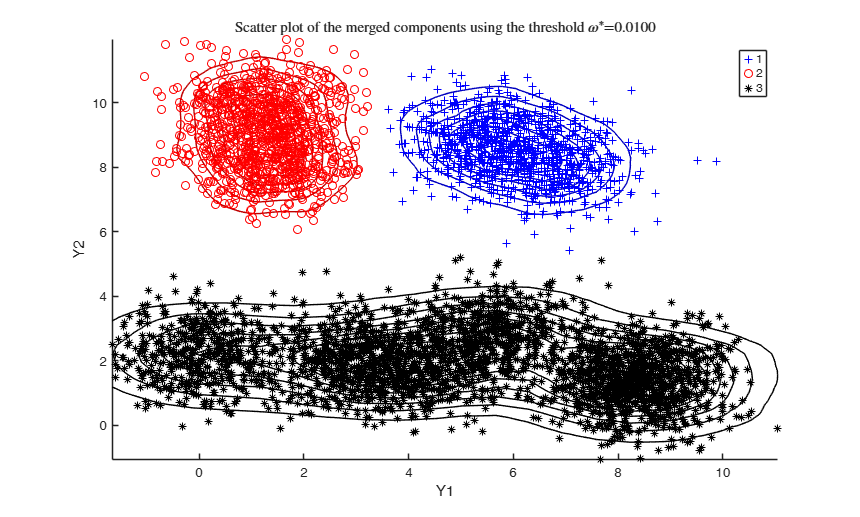###Example using dempk with hierarchical merging on data obtained by simdataset, specifying additional arguments in the call to tkmeans.

close all
% Specify k cluster in v dimensions with n obs
g = 3;
v = 2;
n = 5000;
% null trimming and noise level
alpha0 = 0;
% restriction factor
restr = 30;
% Maximum overlap
maxOm = 0.005;
% Generate heterogeneous and elliptical clusters
rng(500, 'twister');
out = MixSim(g, v, 'sph', false, 'restrfactor', restr, 'int', [0 10], ...
'Display', 'off', 'MaxOmega', maxOm, 'Display','off');
% Simulating data
[X, id] = simdataset(n, out.Pi, out.Mu, out.S);
% Plotting data
gg = gscatter(X(:,1), X(:,2), id);
str = sprintf('Simulated data with %d groups in %d dimensions and %d \nunits, with restriction factor %d and maximum overlap %.2f', ...
g, v, n, restr, maxOm);
title(str,'Interpreter','Latex', 'fontsize', 12);
set(findobj(gg), 'MarkerSize',10);
legend1 = legend(gca,'show');
set(legend1,'LineWidth',1,'Interpreter','latex','FontSize',14, 'Location', 'northwest')
% number of components searched by tkmeans
k = g * 6;
tkmeansOpt = struct;
tkmeansOpt.reftol = 0.0001;
tkmeansOpt.msg = 1;
tkmplots = struct;
tkmplots.type = 'contourf';
tkmplots.cmap = [0.3 0.2 0.4; 0.4 0.5 0.5; 0.1 0.7 0.9; 0.5 0.3 0.8; 1 1 1];
tkmeansOpt.plots = tkmplots;
tkmeansOpt.nomes = 0;
% saving tkmeans output
tkmeansOut = 1;
DEMP = dempk(X, k, g, 'tkmeansOpt', tkmeansOpt, 'plots', 'ellipse');
cascade;
Total estimated time to complete trimmed k means:  0.48 seconds
------------------------------
Warning: Number of subsets without convergence equal to 21.6667%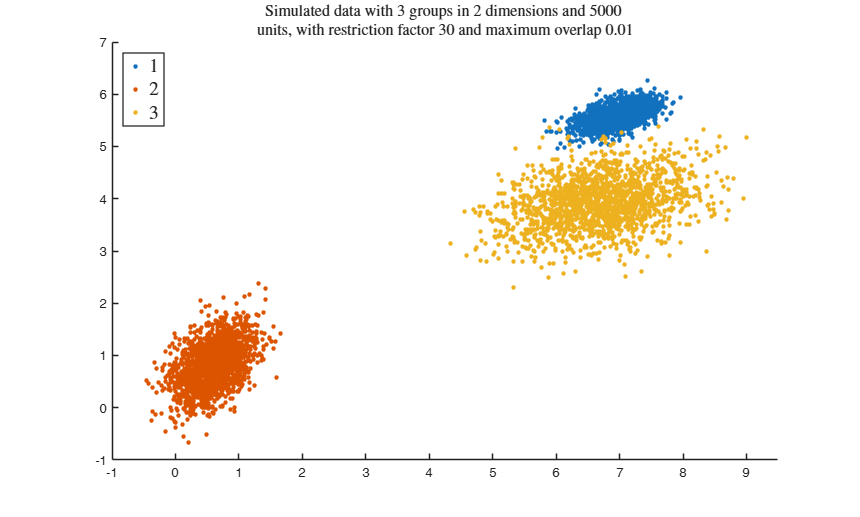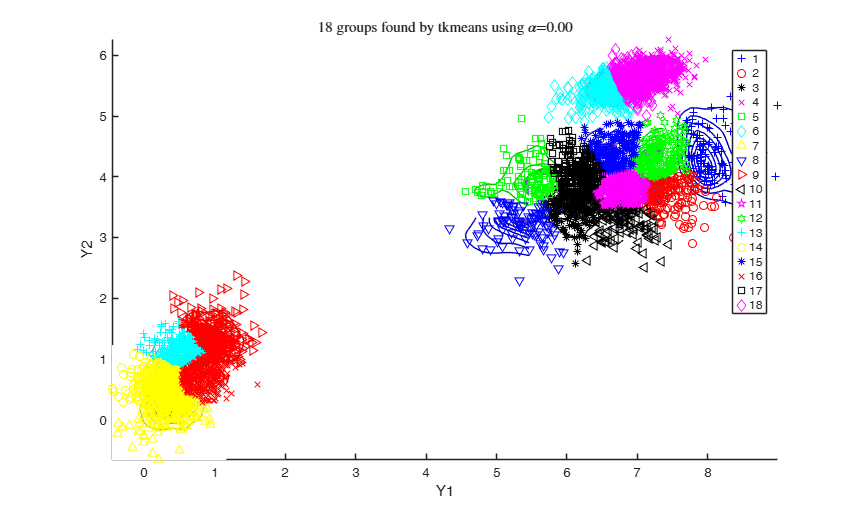## Related Examples

expand all

###Example using dempk with hierarchical merging on data obtained by simdataset, specifying additional arguments in the call to clusterdata.

close all
% Specify k cluster in v dimensions with n obs
g = 3;
v = 2;
n = 5000;
% null trimming and noise level
alpha0 = 0;
% restriction factor
restr = 30;
% Maximum overlap
maxOm = 0.005;
% Generate heterogeneous and elliptical clusters
rng(500, 'twister');
out = MixSim(g, v, 'sph', false, 'restrfactor', restr, 'int', [0 10], ...
'Display', 'off', 'MaxOmega', maxOm, 'Display','off');
% Simulating data
[X, id] = simdataset(n, out.Pi, out.Mu, out.S);
% Plotting data
gg = gscatter(X(:,1), X(:,2), id);
str = sprintf('Simulated data with %d groups in %d dimensions and %d \nunits, with restriction factor %d and maximum overlap %.2f', ...
g, v, n, restr, maxOm);
title(str,'Interpreter','Latex', 'fontsize', 12);
set(findobj(gg), 'MarkerSize',10);
legend1 = legend(gca,'Group 1','Group 2','Group 3');
set(legend1,'LineWidth',1,'Interpreter','latex','FontSize',12, 'Location', 'northwest')
% number of components searched by tkmeans
disp('RUNNING TKMEANS WITH 18 COMPONENTS; THEN MERGING WITH dempk');
k = g * 6;
% additional input for clusterdata (i.e. hierOpt)
cascade;
RUNNING TKMEANS WITH 18 COMPONENTS; THEN MERGING WITH dempk
Total estimated time to complete trimmed k means:  0.36 seconds
------------------------------
Warning: Number of subsets without convergence equal to 37.3333%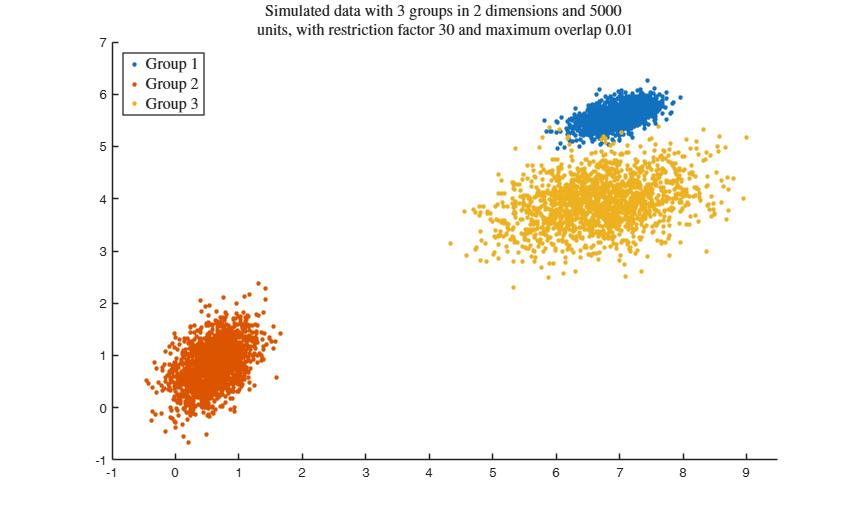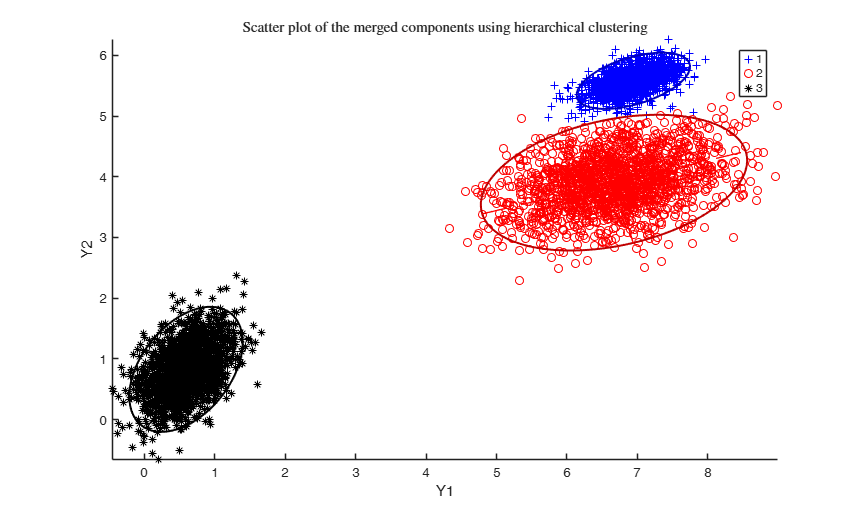###Example using dempk, both setting a threshold and performing a hierarchical merging, for data obtained by simdataset with 10 percent uniform noise.

close all
% Specify k cluster in v dimensions with n obs
g = 3;
v = 2;
n = 5000;
% 10 percent trimming and uniform noise
alpha = 0.1;
noise = alpha*n;
% restriction factor
restr = 30;
% Maximum overlap
maxOm = 0.005;
% Generate heterogeneous and elliptical clusters
rng(500, 'twister');
out = MixSim(g, v, 'sph', false, 'restrfactor', restr, 'int', [0 10], ...
'Display', 'off', 'MaxOmega', maxOm, 'Display','off');
% Simulating data
[X,id] = simdataset(n, out.Pi, out.Mu, out.S, 'noiseunits', noise);
% Plotting data
gg = gscatter(X(:,1), X(:,2), id);
str = sprintf('Simulating %d groups in %d dimensions and %d units with %d%s \nuniform noise, setting a restriction factor %d and maximum overlap %.2f', ...
g, v, n, alpha*100, '\%', restr, maxOm);
title(str,'Interpreter','Latex', 'fontsize', 10);
set(findobj(gg), 'MarkerSize',10);
legend1 = legend(gca,'Outliers','Group 1','Group 2','Group 3');
set(legend1,'LineWidth',1,'Interpreter','latex','FontSize',12, 'Location', 'northwest')
% fixing the number of components searched by tkmeans
k = g * 6;
% dempk with hierarchical merging and trimming equal to the level of noise
DEMP = dempk(X, k, g, 'alpha', alpha, 'plots', 'contourf');
% dempk with a threshold value and trimming equal to the level of noise
g = 0.025;
DEMP = dempk(X, k, g, 'alpha', alpha, 'plots', 'contourf');
cascade;
Total estimated time to complete trimmed k means:  5.87 seconds
------------------------------
Warning: Number of subsets without convergence equal to 38%
Total estimated time to complete trimmed k means:  6.02 seconds
------------------------------
Warning: Number of subsets without convergence equal to 36%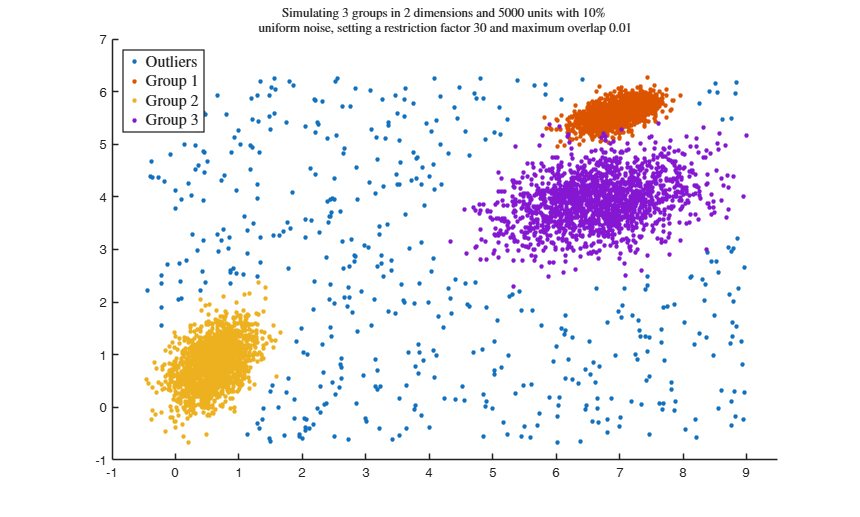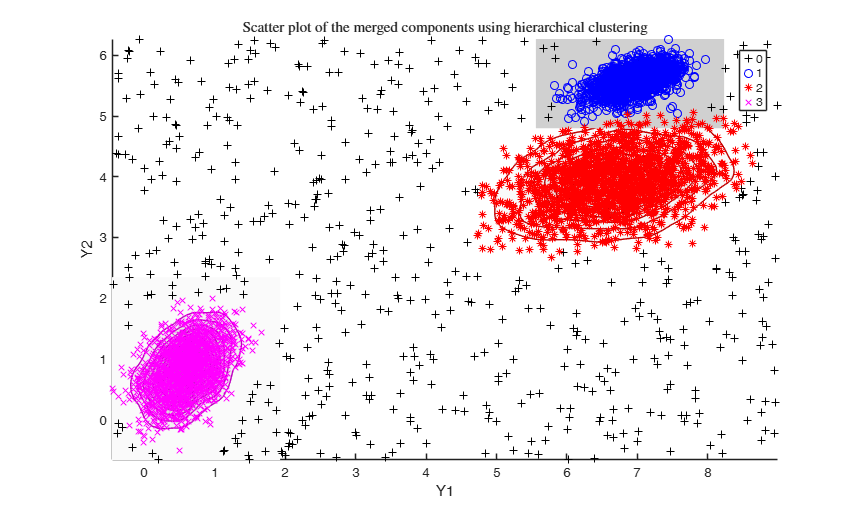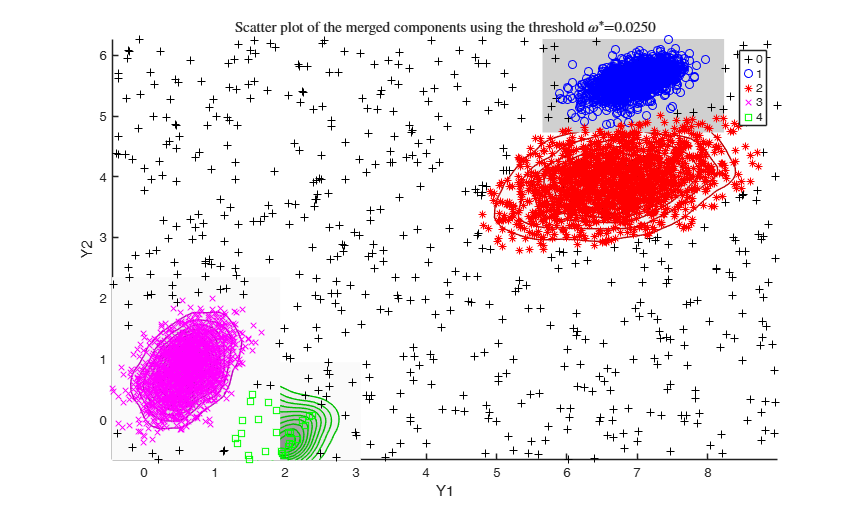###Example using the M5 dataset and various setting for dempk, using hierarchical clustering, in order to identify the real clusters using different strategies.

close all
id = Y(:,3);
Y = Y(:, 1:2);
G = max(id);
n = length(Y);
noise = length(Y(id==0, 1));
v = 2; % dimensions
id(id==0) = -1; % changing noise label
gg = gscatter(Y(:,1), Y(:,2), id);
str = sprintf('M5 data set with %d groups in %d dimensions and \n%d units where %d%s of them are noise', G, v, n, noise/n*100, '\%');
title(str,'Interpreter','Latex', 'fontsize', 12);
set(findobj(gg), 'MarkerSize',12);
legend1 = legend(gca,'Outliers','Group 1','Group 2','Group 3');
set(legend1,'LineWidth',1,'Interpreter','latex','FontSize',12, 'Location', 'northwest')
% number of components to search
k = G*5;
% null trimming and noise level
alpha0 = 0;
% mimimum overlap cut-off value between pair of merged components
omegaStar = 0.045;
DEMP = dempk(Y, k, G, 'alpha', alpha0, 'tkmeansOut', 1, 'plots', 1);
% setting alpha equal to noise level (usually not appropriate)
alpha = noise/n;
DEMP2 = dempk(Y, k, G, 'alpha', alpha, 'tkmeansOut', 1, 'plots', 1);
% setting alpha greater than the noise level (almost always appropriate)
out = dempk(Y, k, G, 'alpha', alpha+0.04, 'tkmeansOut', 1, 'plots', 1);
cascade;
Total estimated time to complete trimmed k means:  0.20 seconds
------------------------------
Warning: Number of subsets without convergence equal to 36%
Total estimated time to complete trimmed k means:  3.21 seconds
------------------------------
Warning: Number of subsets without convergence equal to 39%
Total estimated time to complete trimmed k means:  1.99 seconds
------------------------------
Warning: Number of subsets without convergence equal to 38.6667%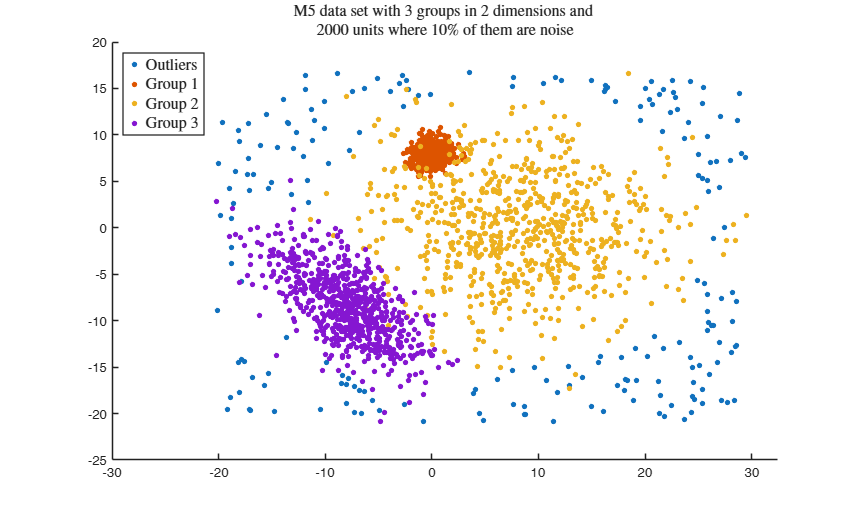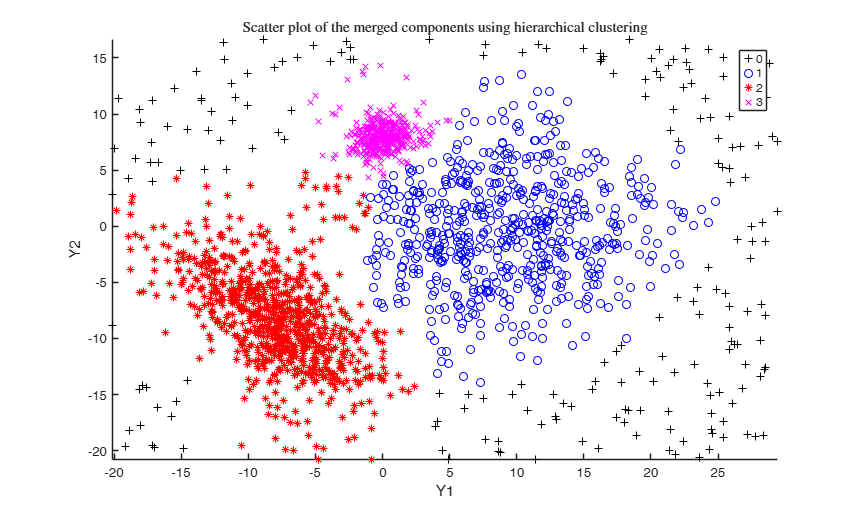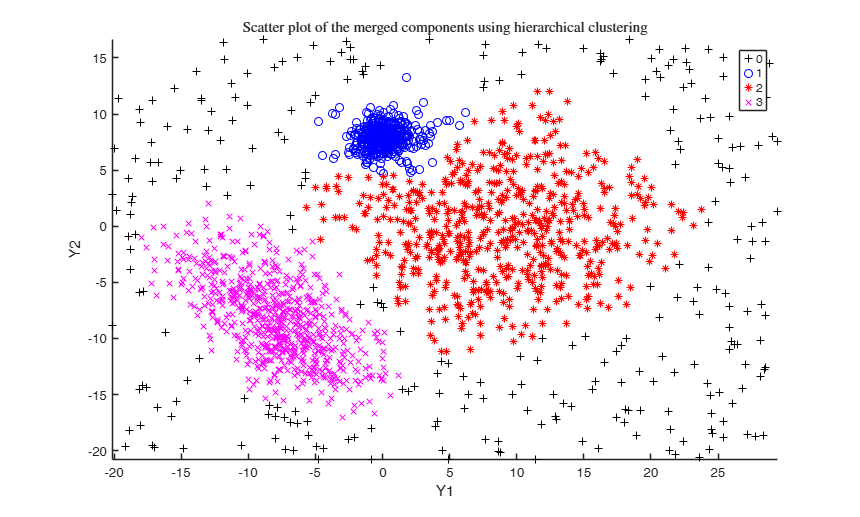## Input Arguments

### Y — Input data. Matrix.

n x v data matrix. n observations and v variables. Rows of Y represent observations, and columns represent variables. Missing values (NaN's) and infinite values (Inf's) are allowed, since observations (rows) with missing or infinite values will automatically be excluded from the computations.

Data Types: single | double

### k — Number of components searched by tkmeans algorithm. Integer scalar.

Data Types: single | double

### g — Merging rule. Scalar.

Number of groups obtained by hierarchical merging, or threshold of the pairwise overlap values (i.e. omegaStar) if 0<g<1.

Data Types: single | double

### Name-Value Pair Arguments

Specify optional comma-separated pairs of Name,Value arguments. Name is the argument name and Value is the corresponding value. Name must appear inside single quotes (' '). You can specify several name and value pair arguments in any order as  Name1,Value1,...,NameN,ValueN.

Example:  'alpha', 0.05 , 'plots', 1 , 'tkmeansOpt.reftol', 0.0001 , 'tkmeansOut', 1 ,, 'Ysave',1 

### alpha —Global trimming level.scalar.

alpha is a scalar between 0 and 0.5. If alpha=0 (default) tkmeans reduces to kmeans.

Example:  'alpha', 0.05 

Data Types: single | double

### plots —Plot on the screen.scalar | char | struct.

- If plots=0 (default) no plot is produced.

- If plots=1, the components merged are shown using the spmplot function. In particular: * for v=1, an histogram of the univariate data.

* for v=2, a bivariate scatterplot.

* for v>2, a scatterplot matrix.

When v>=2 plots offers the following additional features (for v=1 the behaviour is forced to be as for plots=1): - plots='contourf' adds in the background of the bivariate scatterplots a filled contour plot. The colormap of the filled contour is based on grey levels as default.

This argument may also be inserted in a field named 'type' of a structure. In the latter case it is possible to specify the additional field 'cmap', which changes the default colors of the color map used. The field 'cmap' may be a three-column matrix of values in the range [0,1] where each row is an RGB triplet that defines one color.

Check the colormap function for additional informations.

- plots='contour' adds in the background of the bivariate scatterplots a contour plot. The colormap of the contour is based on grey levels as default. This argument may also be inserted in a field named 'type' of a structure.

In the latter case it is possible to specify the additional field 'cmap', which changes the default colors of the color map used. The field 'cmap' may be a three-column matrix of values in the range [0,1] where each row is an RGB triplet that defines one color.

Check the colormap function for additional informations.

- plots='ellipse' superimposes confidence ellipses to each group in the bivariate scatterplots. The size of the ellipse is chi2inv(0.95,2), i.e. the confidence level used by default is 95%. This argument may also be inserted in a field named 'type' of a structure. In the latter case it is possible to specify the additional field 'conflev', which specifies the confidence level to use and it is a value between 0 and 1.

- plots='boxplotb' superimposes on the bivariate scatterplots the bivariate boxplots for each group, using the boxplotb function. This argument may also be inserted in a field named 'type' of a structure.

If plots is a struct it may contain the following fields:

Value Description
type

a char specifying the type of superimposition Choices are 'contourf', 'contour', 'ellipse' or 'boxplotb'.

REMARK - The labels<=0 are automatically excluded from the overlaying phase, considering them as outliers.

Example:  'plots', 1 

Data Types: single | double | string

### tkmeansOpt —tkmeans optional arguments.structure.

Empty structure (default) or structure containing optional input arguments for tkmeans.

See tkmeans function.

Example:  'tkmeansOpt.reftol', 0.0001 

Data Types: struct

### tkmeansOut —Saving tkmeans output structure.scalar.

It is set to 1 to save the output structure of tkmeans into the output structure of dempk. Default is 0, i.e. no saving is done.

Example:  'tkmeansOut', 1 

Data Types: single | double

Example:

Data Types:

### Ysave —Saving Y.scalar.

Scalar that is set to 1 to request that the input matrix Y is saved into the output structure out.

Default is 0, i.e. no saving is done.

Example:  'Ysave',1 

Data Types: double

## Output Arguments

### out — description Structure

Structure which contains the following fields

Value Description
PairOver

Pairwise overlap triangular matrix (sum of misclassification probabilities) among components found by tkmeans.

mergID

Label for each unit. It is a vector with n elements which assigns each unit to one of the groups obtained according to the merging algorithm applied.

REMARK - out.mergID=0 denotes trimmed units.

tkmeansOut

Output from tkmeans function. The structure is present if option tkmeansOut is set to 1.

Y

Original data matrix Y. This field is present only if option Ysave is set to 1.

Melnykov, V., Michael, S. (2020), Clustering Large Datasets by Merging K-Means Solutions, Journal of Classification, Vol. 37, pp. 97–123, https://doi.org/10.1007/s00357-019-09314-8

Melnykov, V. (2016), Merging Mixture Components for Clustering Through Pairwise Overlap, "Journal of Computational and Graphical Statistics", Vol. 25, pp. 66-90.